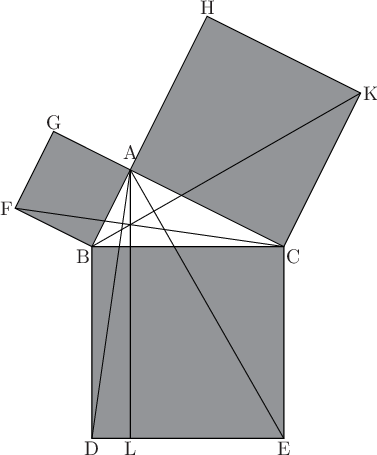## 10.5.3 The construction of large multiplots

By default, whenever an item is added to a multiplot, or an existing item moved or replotted, the whole multiplot is replotted to show the change. This can be a time consuming process on large and complex multiplots. For this reason, the set nodisplay command is provided, which stops Pyxplot from producing any output. The set display command can subsequently be issued to return to normal behaviour.

This can be especially useful in scripts which produce large multiplots. There is no point in producing output at each step in the construction of a large multiplot, and a great speed increase can be achieved by wrapping the script with:

```set nodisplay
[...prepare large multiplot...]
set display
refresh
```

Example: A diagram from Euclid’s Elements

In this more extended example script, we use Pyxplot’s arrow and text commands to reproduce a diagram illustrating the 47th Proposition from Euclid’s First Book of Elements, better known as Pythagoras’ Theorem. A full text of the proof which accompanies this diagram can be found at http://www.gutenberg.org/etext/21076.
set unit angle nodimensionless
set multiplot ; set nodisplay

# Lengths of three sides of triangle
AB = 2*unit(cm)
AC = 4*unit(cm)
BC = hypot(AC, AB) # Hypotenuse
CBA = atan2(AC, AB) # Angle CBA

# Positions of three corners of triangle
Bx = 0*unit(cm)       ; By = 0*unit(cm) # The origin
Cx = Bx + BC          ; Cy = By
Ax = Bx + AB*cos(CBA) ; Ay = By + AB*sin(CBA)

# Positions of constructed points
Dx = Bx               ; Dy = -BC
Lx = Ax               ; Ly = Dy
Ex = Cx               ; Ey = Dy

Hx = Bx + (AB + AC) * cos(CBA)
Hy = By + (AB + AC) * sin(CBA)
Kx = Cx + (     AC) * cos(CBA)
Ky = Cy + (     AC) * sin(CBA)

Fx = Bx + AB*cos(CBA+90*unit(deg))
Fy = By + AB*sin(CBA+90*unit(deg))
Gx = Ax + AB*cos(CBA+90*unit(deg))
Gy = Ay + AB*sin(CBA+90*unit(deg))

# Construct diagram
box from Dx,Dy to Cx,Cy with fillcol gray50
box at Ax,Ay width AC height AC rot CBA-90*unit(deg) with fillcol gray50
box at Bx,By width AB height AB rot CBA with fillcol gray50
line from Bx,By to Kx,Ky
line from Fx,Fy to Cx,Cy
line from Ax,Ay to Dx,Dy
line from Ax,Ay to Lx,Ly
line from Ax,Ay to Ex,Ey

# Label diagram
set fontsize 1.3
TG = 0.5*unit(mm) # Gap left between labels and figure
text "A" at Ax,Ay gap TG*5 hal c val b
text "B" at Bx,By gap TG   hal r val t
text "C" at Cx,Cy gap TG   hal l val t
text "D" at Dx,Dy gap TG   hal c val t
text "E" at Ex,Ey gap TG   hal c val t
text "F" at Fx,Fy gap TG   hal r val c
text "G" at Gx,Gy gap TG   hal c val b
text "H" at Hx,Hy gap TG   hal c val b
text "K" at Kx,Ky gap TG   hal l val c
text "L" at Lx,Ly gap TG   hal c val t

# Display diagram
set display ; refreshExample: A diagram of the conductivity of nanotubes

In this example we produce a diagram of the irreducible wedge of possible carbon nanotube configurations, highlighting those configurations which are electrically conductive. We use Pyxplot’s loop constructs to automate the production of the hexagonal grid which forms the basis of the diagram.
basisAngleX =   0*unit(deg)
basisAngleY = 120*unit(deg)
lineLen     =   5*unit(mm)

# Set up a transformation matrix
transformMat = matrix([[sin(basisAngleX),sin(basisAngleY)],[cos(basisAngleX),cos(basisAngleY)] ])
transformMat *= lineLen

subroutine line(p1,p2,lw)
{
line from transformMat*p1 to transformMat*p2 with linewid lw
}

subroutine hexagon(p,lw)
{
call line(p+vector([ 0, 0]),p+vector([ 0,-1]),lw)
call line(p+vector([ 0,-1]),p+vector([ 1,-1]),lw)
call line(p+vector([ 1,-1]),p+vector([ 2, 0]),lw)
call line(p+vector([ 2, 0]),p+vector([ 2, 1]),lw)
call line(p+vector([ 2, 1]),p+vector([ 1, 1]),lw)
call line(p+vector([ 1, 1]),p+vector([ 0, 0]),lw)
}

set multiplot ; set nodisplay

for x=0 to 10
{
for y=0 to x+1
{
p = vector([x+2*y , 2*x+y])
call hexagon(p, ((x-y)%3==0)?4:1)
text ’%d,%d’%(x,y) at transformMat*(p+vector([1,0]))hal cen val cen
}
}

set display ; refresh